Courses

# Previous Year Questions: Motion in a Straight Line

## 9 Questions MCQ Test Physics Class 11 | Previous Year Questions: Motion in a Straight Line

Description
This mock test of Previous Year Questions: Motion in a Straight Line for JEE helps you for every JEE entrance exam. This contains 9 Multiple Choice Questions for JEE Previous Year Questions: Motion in a Straight Line (mcq) to study with solutions a complete question bank. The solved questions answers in this Previous Year Questions: Motion in a Straight Line quiz give you a good mix of easy questions and tough questions. JEE students definitely take this Previous Year Questions: Motion in a Straight Line exercise for a better result in the exam. You can find other Previous Year Questions: Motion in a Straight Line extra questions, long questions & short questions for JEE on EduRev as well by searching above.
QUESTION: 1

### In 1.0 sec. a particle goes from point A to point B moving in a semicircle of radius 1.0 m. The magnitude of average velocity is -[JEE `99]

Solution:

Total displacement occurred = 2m
Total time time taken = 2sec
Thus average velocity = 2m/s

QUESTION: 2

### A ball is dropped vertically from a height d above the ground it hits the ground and bounces up vertically to a height d/2. Neglecting subsequent motion and air resistances, its velocity v varies with the height h above the ground as -    [JEE' 2000 (Scr)]

Solution: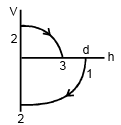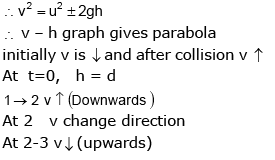QUESTION: 3

### An object A is kept fixed at the point x = 3 m and y = 1.25 m on a plank P raised above the ground. At time t = 0 the plank starts moving along the +x direction with an acceleration 1.5 m/s2. At the same instant a stone is projected from the origin with a velocity u as shown. A stationary person on the ground observes the stone hitting the object during its downward motion at an angle of 45° to the horizontal. All the motions are in x-y plane. Find u and the time after which the stone hits the object. Take g = 10 m/s2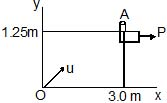[JEE 2000]

Solution: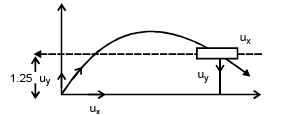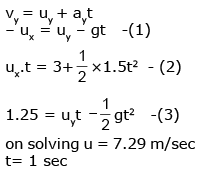QUESTION: 4

On a frictionless horizontal surface, assumed to be the x-y plane, a small trolley A is moving along a straight line parallel to the y-axis (see figure) with a constant velocity of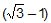m/s. At a particular instant, when the line OA makes an angle of 45° with the x-axis, a ball is thrown along the surface from the origin O. Its velocity makes an angle f with the x-axis and it hits the trolley.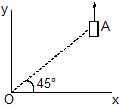(a) The motion of the ball is observed from the frame of trolley. Calculate the angle q made by the velocity vector of the ball with the x-axis in this frame.

(b) Find the speed of the ball with respect to the surface, if f =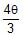.

[JEE 2002]

Solution: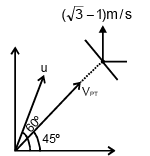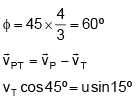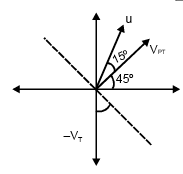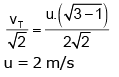QUESTION: 5

A particle starts from rest. Its acceleration (a) varsus time (t) is as shown in the figure. The maximum speed of the particle will be -    [JEE' 2004 (Scr)]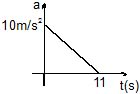Solution:

We know that v is the integral of acceleration wrt time and thus we can say graphically velocity is equal to the area under the acceleration-time graph. Also if we extend the graph the curve would go in a negative zone resulting in producing a negative area under the curve and hence decreasing the magnitude of speed. Hence the maximum speed is achieved when a gets 0. Thus maximum speed is ½ x 10 x 11 = 55m/s

QUESTION: 6

A small block slides without friction down an inclined plane starting from rest. Let Sn be the distance travelled from time t = n-1 to t = n. Then Sn/Sn+1 is-    [JEE' 2004 (Scr)]

Solution:

S=ut+0.5at2= 0.5at2   (since u=0)

At t=n-1

S= 0.5a(n-1)2

At t=n

S'=0.5a(n)2

Sn= S'-S =0.5 [n2 - (n-1)2] = 0.5a (n-n+1)*(n+n-1)

Sn= 0.5a*(2n-1)

put n+1 in place of n in expression of Sn

Sn+1= 0.5a (2(n+1)-1)= 0.5a (2n+1)

Sn/Sn+1 = 2n-1/2n+1

QUESTION: 7

The velocity displacement graph of a particle moving along a straight line is shown. The most suitable acceleration-displacement graph will be -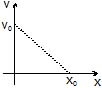Solution: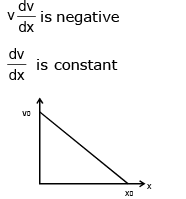but , v is decreasing with x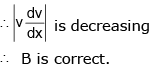QUESTION: 8

Statement-1

For an observer looking out through the window of a fast moving train, the nearby objects appear to move in the opposite direction to the train, while the distant objects appear to be stationary.

Statement-2

If the observer and the object are moving at velocities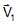and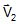respectively with reference to a laboratory frame, the velocity of the object with respect to the observer is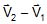[JEE' 2008]

Solution: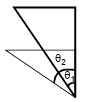θ2 > θ1

QUESTION: 9

A train is moving along a straight line with a constant acceleration 'a'. A boy standing in the train throws a ball forward with a speed of 10 m/s, at an angle of 60° to the horizontal. The boy has to move forward by 1.15 m inside the train to catch the ball back at the initial height. The acceleration of the train in m/s2 is                                                                                                                                                          [JEE' 2011]

Solution: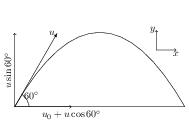Consider the motion in a frame attached to the ground. Let train's velocity at the time of projection of ball be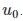The ball is projected with a velocity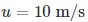(relative to the train) at an angle 60º from the horizontal (see figure). Thus the horizontal and vertical component of the initial velocity of the ball are,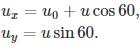The time of flight is given by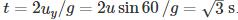The distance travelled by the train and the ball in time t are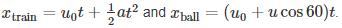Since the boy moves forward by 1.15 m, we have,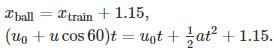Substitute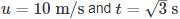in first equation and then solve to get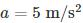. .